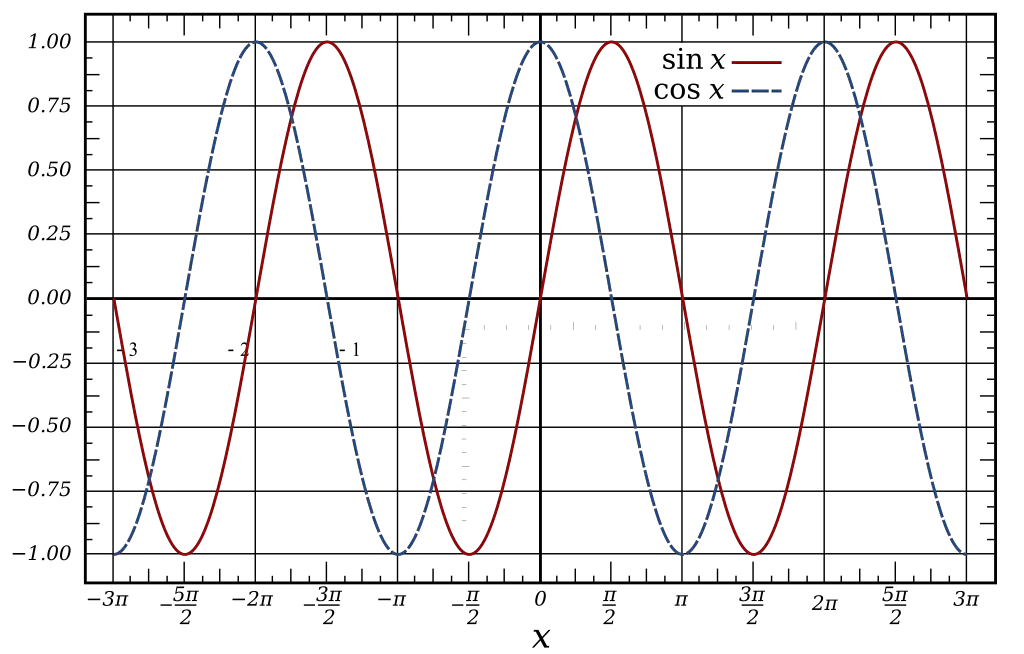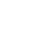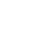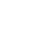# Properties of Waves

The study of waves in physics is extremely critical because it is a means to understand how energy is transported and distributed in the universe. Waves exist all around and there are many types such as that of water, sound, and light.

They are defined by a continuous movement that transports energy from one location to the next.

The movement of energy is defined by the term Oscillation, which means regular movement from up and down or right to left. As the wave transports energy through oscillation, each of these movements are known as a Pulse.When learning about waves, it is important to note that there are two main types of waves: Mechanical and Electromagnetic.

Mechanical waves travel through vibrations or oscillations that are possible through a medium such as water or sound.  The second main type is called Electromagnetic waves, which are made of electric and magnetic fields and travel through light.

Mechanical waves also have 2 types: Transverse and Longitudinal. The first type of Mechanical wave is called Transverse and the transfer of energy vibrates perpendicularly at a 90° (degree) angle to the direction that the energy is moving.

On the other hand, Longitudinal is where the vibrations or oscillations move parallel to the direction that the wave of energy is travelling.

A great example of Longitudinal waves is sound. When people speak, the noise they make causes air particles to vibrate on and on until the noise reaches another person’s ear drums. The vibrating air speeds away from the source, creating a sound wave.## Interesting Facts about the Properties of Waves

• Wavelength is characterized by the symbol (λ) and is the distance between consecutive corresponding points, which means one after the other. The measurement of the Wavelength of waves is in meters.
• Amplitude is characterized by the symbol (A) and considered the height of a wave where the measurement begins from the middle to its top.
• Frequency is characterized by the symbol (f) and is the number of full wavelengths produced in each second. The unit by which a Frequency is measured is known as a Hertz (Hz).
• Period is characterized by the symbol (T) and is the time measured for one complete wavelength to be produced.
• Speed is characterized by the symbol (v) and it represents how quickly a wave transports energy from one location to the next.
• The calculation by which a wave’s speed is determined is by multiplying its wavelength by its frequency.
• Waves are made up of peaks that consist of crests and troughs. The crests are the bumps found on top of the wave while the troughs are the bumps found at the bottom of the wave.
• A soundwave travelling through the speed of sound does not depend on how loud its sound but if it is travelling through air or water.

### How to calculate the Frequency of a wave?

The Frequency of a wave determines the number of cycles that occur per second. To properly calculate the frequency of a wave requires taking the number of cycles and dividing them by the time of each wave.

### How to calculate the Period of a wave?

The Period is known as the time it takes to complete one cycle. To properly calculate the period of a wave requires taking the total time of a wave and dividing it by the number of cycles that occur.

### What are examples of Transverse waves?

There are many examples of transverse waves that are found in everyday objects. Water waves that are found on the ocean are example of transverse waves as well as a multitude of electromagnetic waves such as:

• Light Waves
•History
•Science
•Geography
•Biography
•US History